### Introduction

In a related tutorial, we described A Robust Method for Measuring Clock Jitter as variation in a clock signal's period. Clock jitter is characterized by the standard deviation (sdev) of the clock period measurement. The track function of the clock period sdev shows us the variations in jitter over time, synchronous with the waveform source.

In this tutorial, we'll make use of the oscilloscope track function to match clock jitter variations to possible sources of jitter. The tutorial will investigate how variations in the voltage level of a power rail, including ripple and noise, affect the clock jitter level. A known variation in the power rail voltage is created using a function generator. The offset voltage of the function generator provides the power to the clock signal source. The square wave output of the function generator is the perturbing signal. We'll measure both the perturbing voltage and resulting clock jitter to determine the clock jitter sensitivity to rail voltage changes.

### Test Equipment

You can perform these procedures in your lab using any clock signal source that is powered by a 5 V rail.

Besides your clock signal source, you will need:

• A real-time oscilloscope capable of sampling at least 5x the signal bandwidth based on the signal rise time (bandwidth = 5*(0.35/trise) for oscilloscopes with single-pole frequency response)

We used a WavePro HD 12-bit, 4-Ch, 8 GHz, 20 GS/s, 5 Gpts oscilloscope with 60 fs sample clock jitter to measure a square wave signal between 10 and 66 MHz. We recommend at least 2-Ch, 2 GHz, 20 Gs/s with track, histogram and low-pass filter functions.

• Function generator , 50 Ω output impedance, 10 kHz square wave, 200 mV amplitude, 10 V offset range
• Clean power source , 5 V DC
• Two 50 Ω coaxial cables to input signals from clock and function generator, or equivalent single-ended probe to input your clock signal

The clock source used in our examples is a 5-stage ring oscillator based on the 74AC14 hex inverter powered by a 5 V rail, shown embedded in a PCB in Figure 2. The logical state of the stage 5 output is inverted from the original stage 1 input, resulting in oscillation with a period equal to 10 times the propagation delay of each hex inverter stage. The specified propagation delay for a power level of 5 V is from 1.5 ns to 10 ns, so the clock period is anywhere in the range of 15 ns to 100 ns, for a frequency anywhere from 66 MHz to 10 MHz. This extreme variation is why it is so important to test clock jitter in situ for every application.

### Step-by-Step

##### Measure Clock Jitter Using a Clean Power Source

1. Recall the oscilloscope's default setup to bring it into a known state.

2. Connect your clock signal source to the clean 5 V power source and an oscilloscope input channel.

3. On the oscilloscope:

a. Set the clock channel to 50 Ω input termination, 1 V/div vertical scale.

b. Set the timebase to 5 ns/division.

c. Set a 50% Edge trigger on the clock channel.

4. Use parameters with statistics on to measure the clock signal rise time, frequency and period.

The display should be similar to Figure 2. This setup shows the period of the clock signal (P3) when measured with a stable power source. The period sdev is a figure of merit for the clock signal jitter.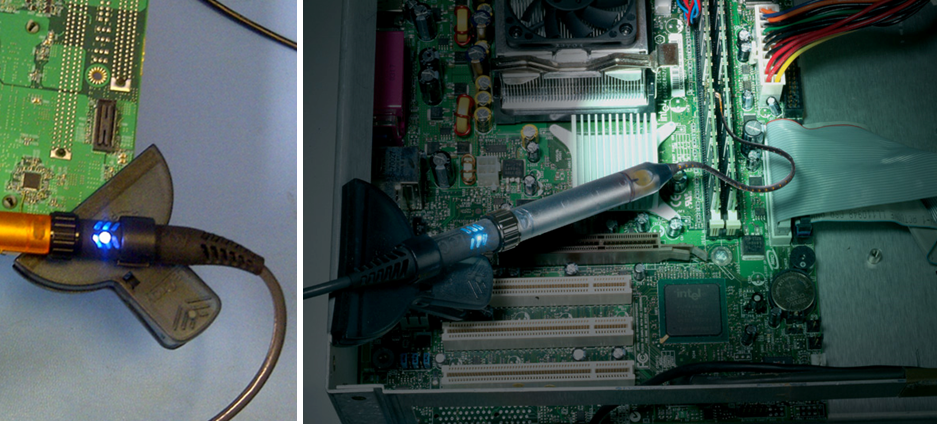Figure 2. Measuring clock period with clock powered by a clean source.
##### Create a Power Source with a Known Voltage Perturbation

5. Connect the output of the function generator to an oscilloscope input channel.

In our examples, C2 (pink) is the clock signal and C3 (blue) is the function generator output.

6. Set the oscilloscope channel that is inputting the function generator to:

a. 1 MΩ input termination

b. 200 mV/div with 5 V offset

7. Set the oscilloscope timebase to 20 µs/div, fixed sampling rate 10 GS/s or greater. We sampled at 20 GS/s.

8. Set the function generator to output a 200 mV peak-to-peak, 10 kHz square wave with a 5 V offset.

9. Connect the function generator's sync output to the oscilloscope's Ext. input.

10. Set a 50% Edge trigger on the Ext. trigger source.

The function generator display should be similar to Figure 3.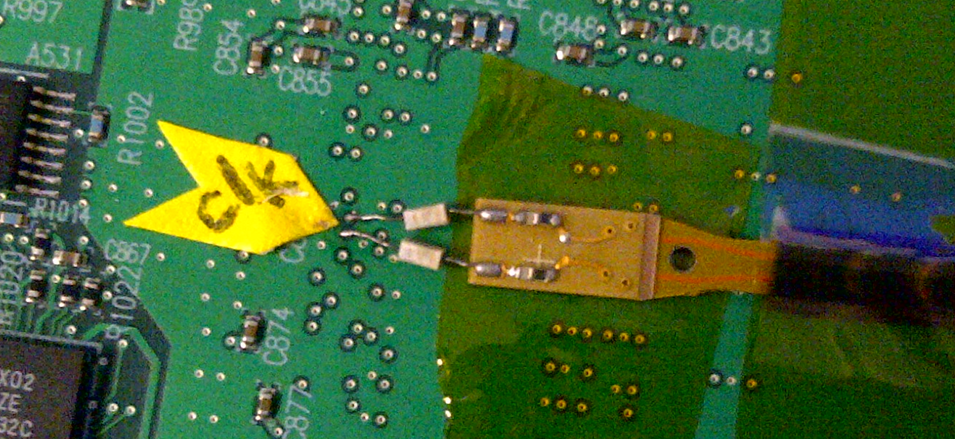Figure 3. The function generator output simulating a 5 V power rail with a small square wave perturbation.
##### Connect the Perturbed Power Source to the Clock

11. While maintaining the connection from the function generator to the oscilloscope, use a coaxial tee adaptor to replace the stable power input to the clock with the function generator output.

Note: From here on, we'll refer to the function generator output as the power source and its trace as the power trace. The power trace will now be off the screen.

12. Readjust the V/div and Offset to get the power trace back on the screen as shown in Figure 4.

13. Apply the (Vertical group) Mean measurement parameter with statistics on to the power trace and read the mean amplitude of the power source.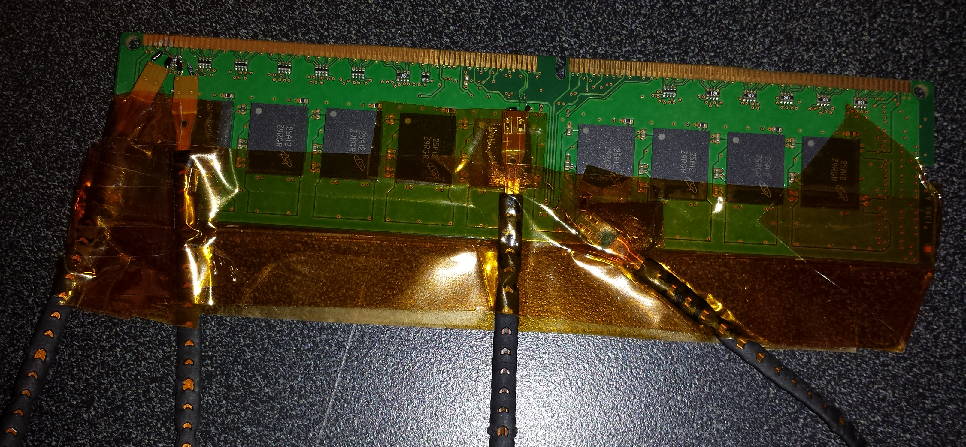Figure 4. The power trace (function generator output) after connecting it to the clock. Note the drop in voltage.

In our example, the mean output level has dropped from 5 to 3.3 V. When the power source is connected to the clock, the voltage decreases significantly. These effects are due to the loading of the power source by the ring oscillator. Think of the power source in terms of its Thevenin equivalent circuit with an output impedance of 50 Ω as in Figure 5.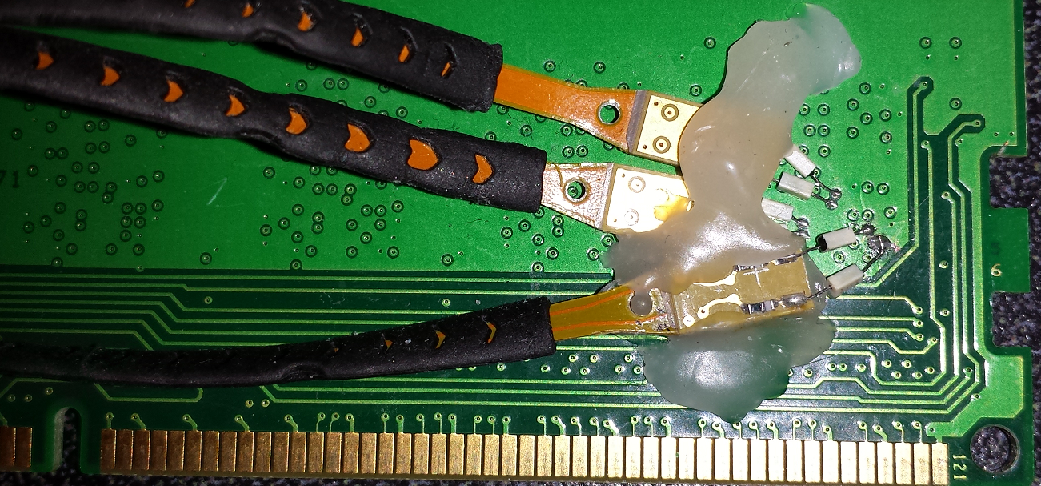Figure 5. Power source Thevenin equivalent output resistance of 50 Ω.

Connecting the clock to the power source forms a voltage divider. Since the impedance of the clock is similar to the 50 Ω output impedance of the power source, the voltage drops.

14. Adjust the offset of the power source until it is again 5 V as read by the mean parameter.

15. Once the 5 V level has been restored:

a. Increase the power source channel vertical Offset to 5 V.

b. Set Vertical Scale to 100 mV/div.

The power trace should have a visible oscillation on it (ours is 400 mV peak-to-peak), as shown in Figure 7.

##### Investigate the Relationship Between the Clock and Power Source

Since this oscillation happened after the power source was connected to the clock, and not before, it is obviously somehow related to the clock. As it happens, the ring oscillator load changes with every output state change, causing the oscillation.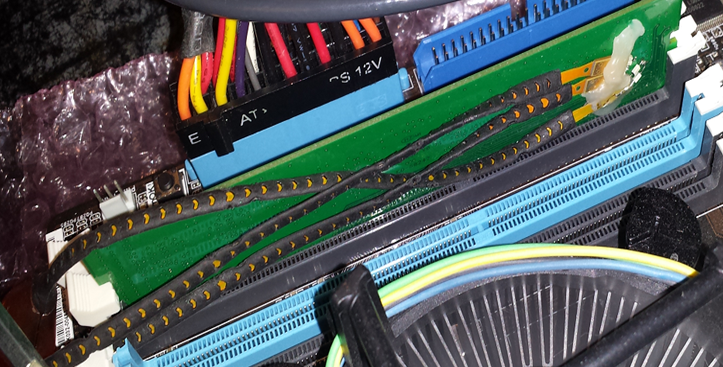Figure 6. 400 mVpp oscillation related to the clock appears on the power trace.

16. Set a 50% Edge trigger on the clock signal.

17. Display both the clock signal trace and and the power trace on the same grid and zoom in until you can see individual cycles of the waveforms, as in Figure 7.

Tip: To do this, just drag the trace descriptor boxes to the same grid.

If the waveforms are synchronous, as shown in Figure 7, we can verify that the oscillation in the power trace is most probably due to the effects of the clock.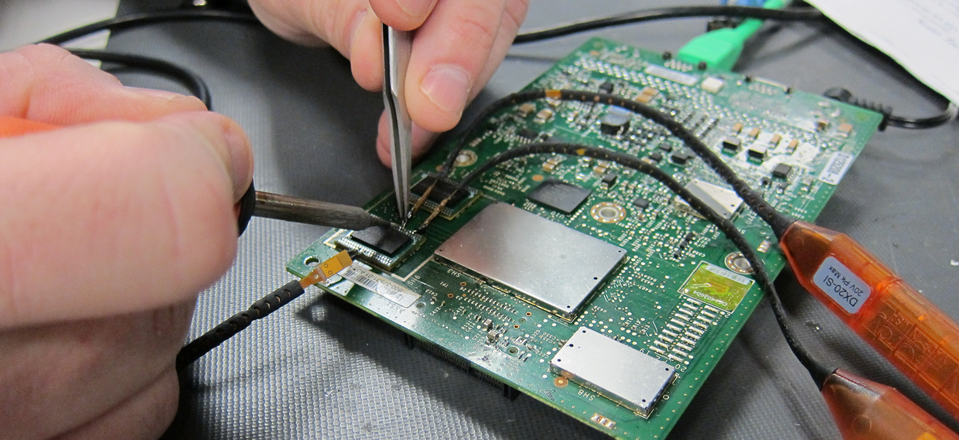Figure 7.The noise on the power rail and the clock output are synchronous. The 48 MHz frequency noise is from the clock.
##### Eliminate the Power Trace Oscillation with Filters

However, at this stage, we can't accurately measure the voltage variation amplitude on our power trace because of the clock noise causing a 400 mVpp oscillation. This clock noise is swamping the 10 kHz perturbation signal and needs to be eliminated before we can measure the voltage variation amplitude. The simplest way to accomplish this is to filter the power trace and conduct our measurements on the filtered signal. The interfering noise has a frequency of 48 MHz, the perturbation frequency is 10 kHz, so a low-pass filter with a cutoff frequency of about 1 MHz should remove the 48 MHz oscillation.

18. Create a math function using your choice of low-pass filter operator and the power source channel.

We applied a 4th order Butterworth low-pass filter with a cutoff frequency of 1 MHz to C3.

Note: This filter is made available with the installation of the Digital Filter Package (DFP2) option on the oscilloscope. However, other options make similar filters available, and they can be used, as well.

19. Set a 50% Edge trigger on the power source channel.

20. View the filter function on the oscilloscope display:

a. Set 20 µs/div horizontal scale.

b. Set to 20mV/div vertical scale, as seen in Figure 8.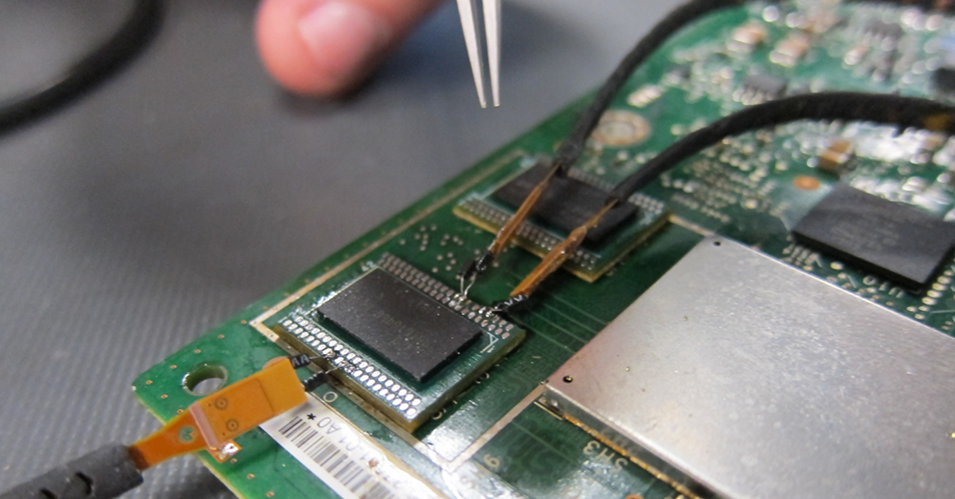Figure 8. Low-pass filter on the power rail signal removes the high-frequency oscillation and allows observation of the 10 kHz perturbing signal.
##### Situational Awareness: Understand Effect of Circuit Design on Power Trace

Before we connected the power source to the clock, our example power trace was a 10 kHz square wave with a 200 mV peak-to-peak amplitude. Now, the signal appears to be a triangle wave of 10 kHz, but with an amplitude of about 20 mV. What happened?

The answer can be found by observing the design of our circuit board. There is a 10 µF bypass capacitor between the 5 V power rail and ground, shown in Figure 9.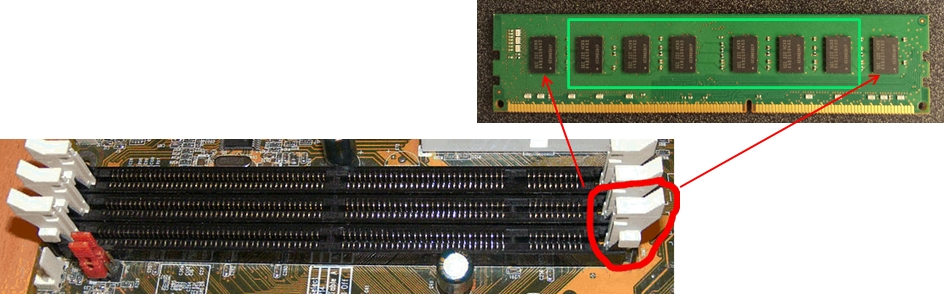Figure 9. A 10 µF bypass capacitor between the 5 V rail and ground effectively low-pass filters the power rail.

The 10 µF capacitor loading the 50 Ω power source forms a low-pass filter with a cutoff frequency of 318 Hz. The low-pass filter is an integrator. The RC combination has a time constant of 500 µs, so the 10 kHz waveform has a positive half cycle period of less than one tenth of the time constant. No wonder it only rises to 20 mV during the positive half cycle of the square wave. Nevertheless, this waveform, as it is, is adequate to calculate the clock jitter sensitivity to power rail voltage variations, because it has a measurable variation in the power rail voltage and a related jitter response, which are key to making our calculation.

##### Calculate the Clock Jitter Sensitivity to Rail Voltage

21. Increase the vertical scale of the power trace to 20 mV/div.

22. Turn on the track of the clock period measurement (for our purposes, the jitter track). You can do this by touching the Track button at the bottom of the period parameter set up dialog.

23. Set the track vertical scale to 50 ps/div, as shown in Figure 10.

24. Move the clock period (jitter) track onto the same grid as the power trace.

25. Increase the amplitude of the offset power (function generator output) until the perturbing signal amplitude on the power trace is about 4 divisions (80 mV).

When we compare the traces, it is obvious that they are related. Both are synchronous with the trigger on the power source. The clock jitter track is inverse to the power rail voltage variation because the propagation delay of the inverters in the ring oscillator decreases as the power rail voltage increases, raising the oscillator frequency and lowering its period.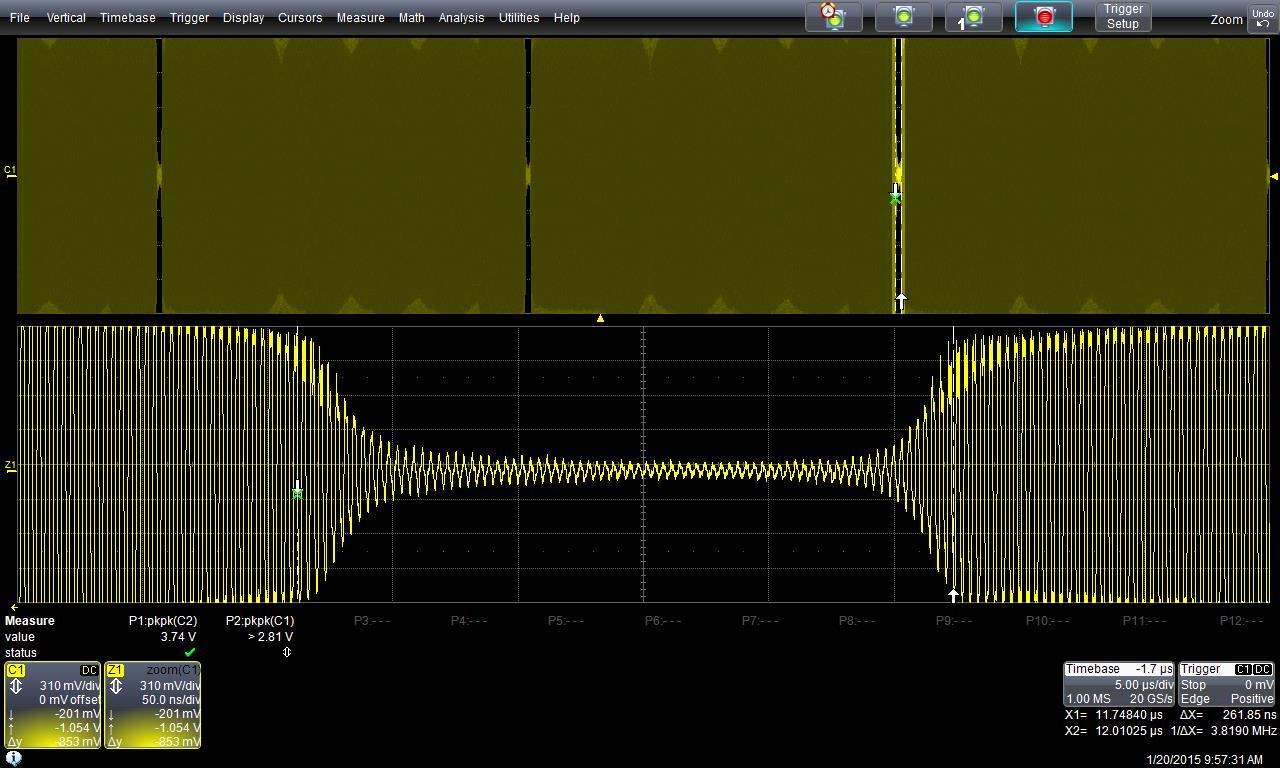Figure 10. The voltage variations on the power rail shown in the same grid as the clock period track (jitter track). These variations form the basis of the clock jitter sensitivity measurement.

The sensitivity of the clock period jitter to the voltage variations on the power rail can be calculated by taking the ratio of their peak-to-peak amplitudes.

In our example, the clock jitter variation is about 150 ps while that of the power rail voltage is about 80 mv. Taking their ratio, the clock jitter sensitivity is about 1.85 ps/mV, rounded up to 2 ps/mV to keep calculations simple.

Now that we know the clock jitter sensitivity to variations of the power rail voltage, we can anticipate the clock period jitter if we know the voltage variation. Likewise, if we measure the clock jitter, we can anticipate the voltage variation that produced it.

##### Verify Clock Jitter Sensitivity to Rail Voltage Variations

This measured sensitivity to voltage variations can be verified by repeating the measurement using a different power source. When we exchange the power supply connected to the clock to a switched-mode power supply, we get a different instance of power rail voltage variations and related clock jitter, shown in Figure 10.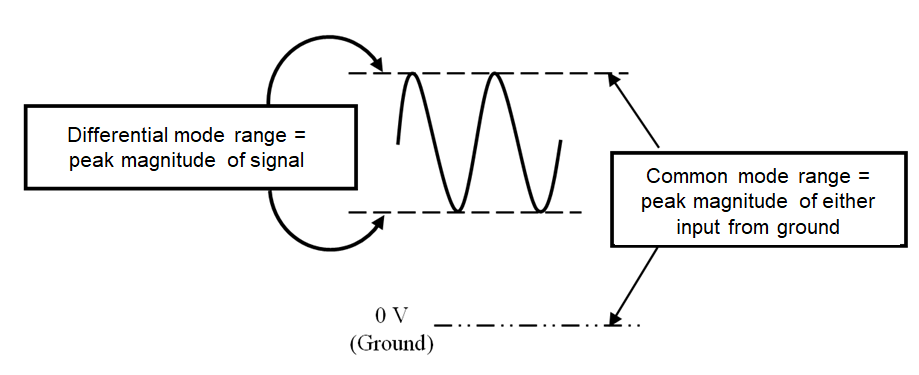Figure 10: Switching the power supply verifies our clock jitter sensitivity measurement. Note that the jitter scaling has been appended at the right for convenience.

This power supply (upper trace) has voltage variations of about the same 10 kHz frequency as did our function generator. In this case, its due to the switching frequency. The estimated amplitude of the clock jitter track (lower trace), ignoring the random noise, is about 20 ps. Given the clock jitter sensitivity we calculated at about 2 ps/mV, we can estimate the power rail voltage variation should be about 10 mV. Looking at the power rail voltage, again ignoring the random noise, we see that the amplitude is indeed about 10 mV.

### Conclusion

By tracking the clock period measurement to obtain the pattern, or the “signature”, of the jitter variation, we can compare that to the fingerprint of other features in our circuit, in this case, the power rail voltage. Taking the ratio of the peak-to-peak amplitudes of the jitter track and the underlying modulation of the power rail, we can calculate the clock jitter sensitivity to voltage variation. Switching from a clean to two different perturbed power sources in the process acts as a consistency check of our findings.

Watch these measurements conducted by Dr. Eric Bogatin in the on-demand webinar, The Impact of Power Rail Noise on Clock Jitter .”